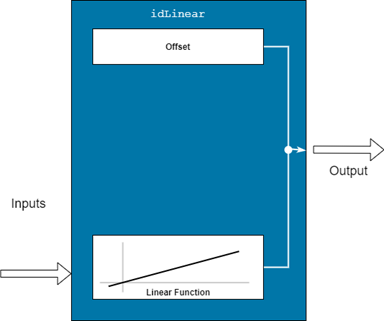idLinear

Linear mapping object for nonlinear ARX models

Description

An idLinear object implements an affine function, and is a mapping function for estimating nonlinear ARX models. The mapping function uses a combination of linear weights and an offset. Unlike the other mapping objects for the nonlinear models, the idLinear object contains no accommodation for a nonlinear component.Mathematically, idLinear is a linear function $y=F\left(x\right)$ that maps m inputs X(t) = [x(t1),x2(t),…,xm(t)]T to a scalar output y(t). . F is a (affine) function of x:

$y\left(t\right)={y}_{0}+{\left(Χ\left(t\right)-\overline{X}\right)}^{T}PL$

Here:

• X(t) is an m-by-1 vector of inputs, or regressors, with mean $\overline{Χ}$.

• y0 is the output offset, a scalar.

• P is an m-by-p projection matrix, where m is the number of regressors and is p is the number of linear weights. m must be greater than or equal to p.

• L is a p-by-1 vector of weights.

Set idLinear as the value of the OutputFcn property of an idnlarx model. For example, specify idLinear when you estimate an idnlarx model with the following command.

sys = nlarx(data,regressors,idLinear)
When nlarx estimates the model, it also estimates the parameters of the idLinear function.

Use the idLinear mapping object when you want to create nonlinear ARX models that operate linearly on the regressors. The regressors themselves can be nonlinear functions of the inputs and outputs. The polynomialRegressor and customRegressor commands allow you to create such regressors. When the idnlarx model has no custom regressors and the output function is set to idLinear, the model is similar to a linear ARX model. However, for the nonlinear ARX model, the offset is an estimable parameter.

You can configure the idLinear object to disable components and fix parameters. Use evaluate to compute the output of the function for a given vector of inputs.

Creation

Description

example

Lin = idLinear creates an idLinear object Lin with unknown parameters.

Properties

expand all

Input signal information for signals used for estimation, specified as vectors of m property-specific values, where m is the number of input signals. The Input properties for each input signal are as follows:

• Name — Names of the input signals, specified as a 1-by-m string or character array, where m is the number of inputs

• Mean — Mean of the input signals, specified as a numeric scalar

• Range — Ranges of the input signals, specified as a 2-by-m numeric array that contains the minimum and maximum values

Output signal information, specified as property-specific values. The Output properties are as follows:

• Name — Name of the output signal, specified as a string or a character array

• Mean — Mean of the output signal, specified as a numeric scalar

• Range — Range of the output signal, specified as 2-by-1 numeric array that contains the minimum and maximum values.

Parameters of the linear function, specified as follows:

• Value — Value of L', specified as a 1-by-m vector.

• Free — Option to update entries of Value during estimation. specified as a logical scalar. The software honors the Free specification only if the starting value of Value is finite. The default value is true.

• Minimum — Minimum bound on Value, specified as a 1-by-p vector. If Minimum is specified with a finite value and the starting value of Value is finite, then the software enforces that minimum bound during model estimation.

• Maximum — Maximum bound on Value, specified as a 1-by-p vector. If Maximum is specified with a finite value and the starting value of Value is finite, then the software enforces that maximum bound during model estimation.

• SelectedInputIndex — Indices of idLinear inputs (see Input.Name) that are used as inputs to the linear function, specified as an 1-by-nr integer vector, where nr is the number of inputs. The RegressorUsage property of the idnlarx model determines these indices.

Parameters of the offset term, specified as follows:

• Value — Offset value, specified as a scalar.

• Free — Option to update Value during estimation, specified as a scalar logical. The software honors the Free specification of false only if the value of Value is finite. The default value is true.

• Minimum — Minimum bound on Value, specified as a numeric scalar or –Inf. If Minimum is specified with a finite value and the value of Value is finite, then the software enforces that minimum bound during model estimation. The default value is -Inf.

• Maximum — Maximum bound on Value, specified as a numeric scalar or Inf. If Maximum is specified with a finite value and the starting value of Value is finite, then the software enforces that maximum bound during model estimation. The default value is Inf.

Examples

collapse all

Create an idLinear mapping object L.

L = idLinear;

Create model regressors that include nonlinear polynomial regressors.

Reg1 = linearRegressor({'y1','u1'},{1:4, 0:4});
Reg2 = polynomialRegressor({'y1','u1'},{1:2, 0:2},2,false,true,true);
Reg3 = polynomialRegressor({'y1','u1'},{2, 1:3},3,false,true);

Estimate the nonlinear ARX model.

sys = nlarx(z7,[Reg1;Reg2;Reg3],L)
sys =
Nonlinear ARX model with 1 output and 2 inputs
Inputs: u1, u2
Outputs: y1

Regressors:
1. Linear regressors in variables y1, u1
2. Order 2 regressors in variables y1, u1
3. Order 3 regressors in variables y1, u1
List of all regressors

Output function: None
Sample time: 1 seconds

Status:
Estimated using NLARX on time domain data "z7".
Fit to estimation data: 43.22% (prediction focus)
FPE: 5.66, MSE: 4.963

Compatibility Considerations

expand all

Not recommended starting in R2021b

Not recommended starting in R2021a